# Olympiad Test: Figure Matrix - 2

## 15 Questions MCQ Test National Cyber Olympiad Class 6 | Olympiad Test: Figure Matrix - 2

Description
Attempt Olympiad Test: Figure Matrix - 2 | 15 questions in 30 minutes | Mock test for Class 6 preparation | Free important questions MCQ to study National Cyber Olympiad Class 6 for Class 6 Exam | Download free PDF with solutions
QUESTION: 1

Solution:
QUESTION: 2

Solution:
QUESTION: 3

### Directions: In each of the following questions, find out which of the answer figures (A), (B), (C) and (D) completes the figure matrix? Q. Select a suitable figure from the four alternatives to make the figure matrix complete.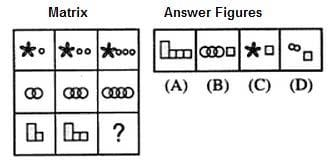Solution:
QUESTION: 4

Directions: In each of the following questions, find out which of the answer figures (A), (B), (C) and (D) completes the figure matrix?

Q. Select a suitable figure from the four alternatives to make the figure matrix complete.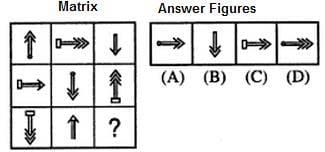Solution:
QUESTION: 5

Directions: In each of the following questions, find out which of the answer figures (A), (B), (C) and (D) completes the figure matrix?

Q. Select a suitable figure from the four alternatives to make the figure matrix complete.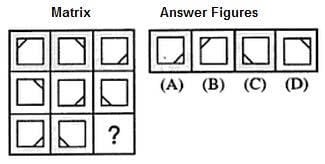Solution:
QUESTION: 6

Directions: In each of the following questions, find out which of the answer figures (A), (B), (C) and (D) completes the figure matrix?

Q. Select a suitable figure from the four alternatives to make the figure matrix complete.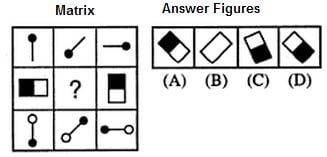Solution:
QUESTION: 7

Directions: In each of the following questions, find out which of the answer figures (A), (B), (C) and (D) completes the figure matrix?

Q. Select a suitable figure from the four alternatives to make the figure matrix complete.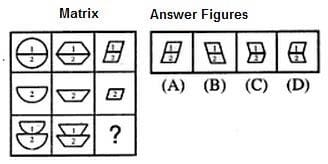Solution:
QUESTION: 8

Directions: In each of the following questions, find out which of the answer figures (A), (B), (C) and (D) completes the figure matrix?

Q. Select a suitable figure from the four alternatives to make the figure matrix complete.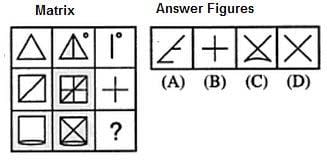Solution:
QUESTION: 9

Directions: In each of the following questions, find out which of the answer figures (A), (B), (C) and (D) completes the figure matrix?

Q. Select a suitable figure from the four alternatives to make the figure matrix complete.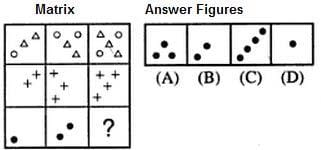Solution:
QUESTION: 10

Directions: In each of the following questions, find out which of the answer figures (A), (B), (C) and (D) completes the figure matrix?

Q. Select a suitable figure from the four alternatives to make the figure matrix complete.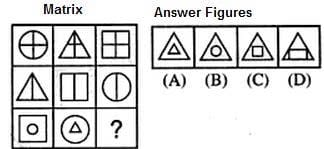Solution:
QUESTION: 11

Directions: In each of the following questions, find out which of the answer figures (A), (B), (C) and (D) completes the figure matrix?

Q. Select a suitable figure from the four alternatives to make the figure matrix complete.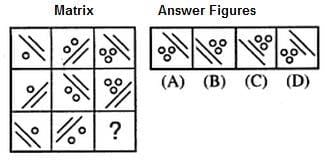Solution:
QUESTION: 12

Directions: In each of the following questions, find out which of the answer figures (A), (B), (C) and (D) completes the figure matrix?

Q. Select a suitable figure from the four alternatives to make the figure matrix complete.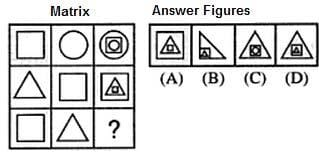Solution:
QUESTION: 13

Directions: In each of the following questions, find out which of the answer figures (A), (B), (C) and (D) completes the figure matrix?

Q. Select a suitable figure from the four alternatives to make the figure matrix complete.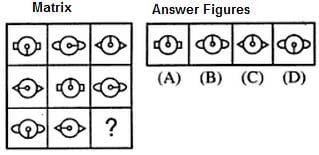Solution:
QUESTION: 14

Directions: In each of the following questions, find out which of the answer figures (A), (B), (C) and (D) completes the figure matrix?

Q. Select a suitable figure from the four alternatives to make the figure matrix complete.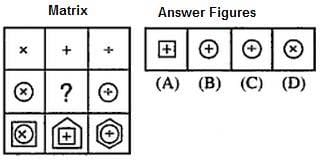Solution:
QUESTION: 15

Directions: In each of the following questions, find out which of the answer figures (A), (B), (C) and (D) completes the figure matrix?

Q. Select a suitable figure from the four alternatives to make the figure matrix complete.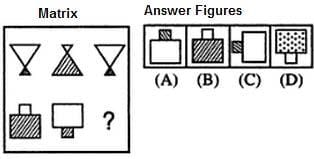Solution:Use Code STAYHOME200 and get INR 200 additional OFF Use Coupon Code